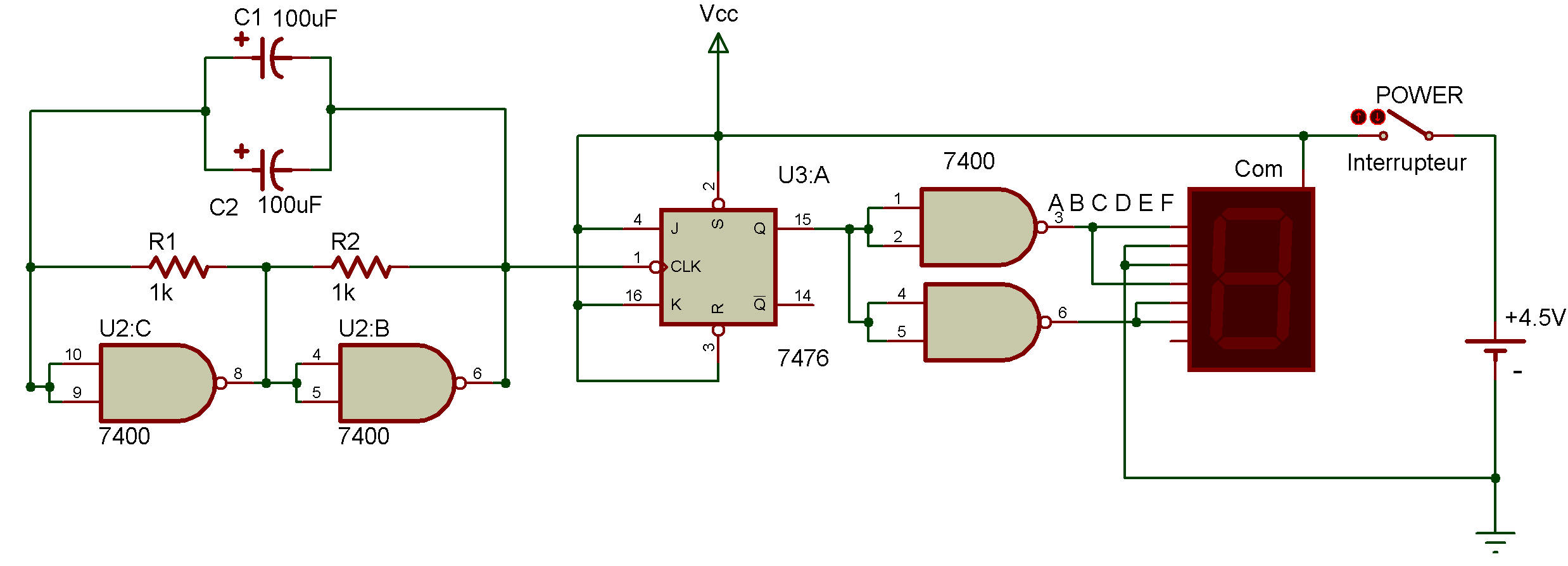Binary counter with display
7400, 7476Counters aren't terribly exciting circuits when they just flash LEDs ... but add the Digital Display and it's a different story altogether.

Here's a binary counter which will let you see it in action as it counts. You will recognize this circuit as being very similar to the binary counter you built back in Project Basic counter circuit.

The source of the pulses for the counter is a NAND multivibrator. The output of the multivibrator provides clock signals for the flip-flop. Note that the output of the flip-flop is taken from output Q and serves as the input for the two NAND gates. (So the two NAND gates aren't really part of a line decoder.)

Put the power ON. You'll see 0 and 1 flash on and off the Display, Why 0 and 1 instead of 1 and 2?

We called this circuit a binary counter... and binary numbers are those made up entirety of 0 and 1 see Binary Number System. 0 and 1 mean the same thing in the binary number system that they do in our normal (called decimal) number system. But what happens when we want to express the number 2 in the binary system?

We can't write "2" since we can only use 0 and 1 in the binary system. We do in this case what we do when we reach. 9 in our decimal system ...we write "10!" That's right, 10 in binary numbers is actually 2 in the decimal system. How do you suppose we write 3 in binary numbers?

Recherche personnalisée